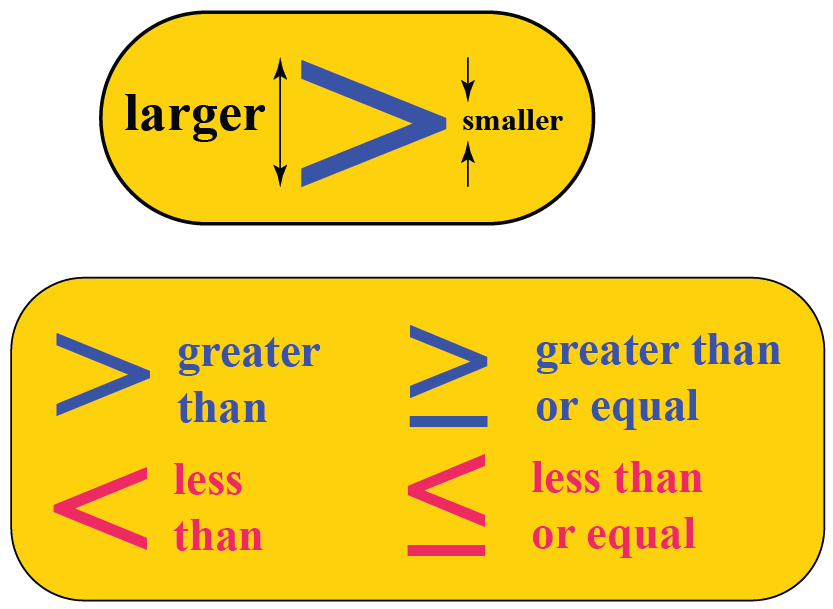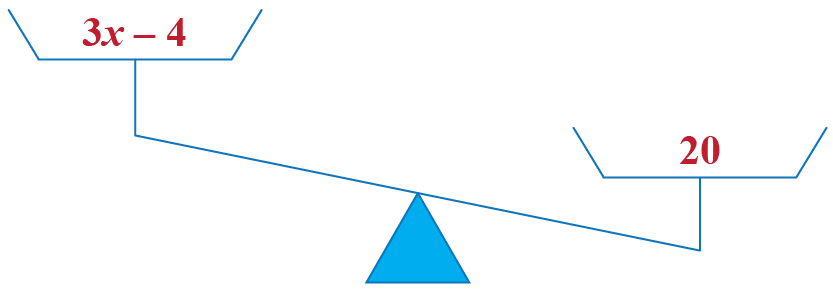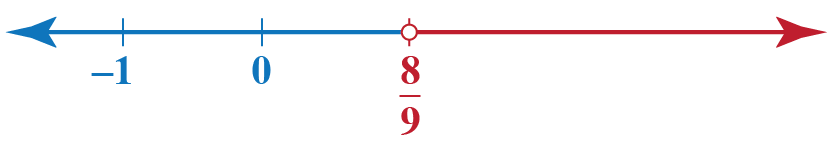# Linear Inequalities

Linear Inequalities
Go back to  'Inequalities'

In this mini-lesson, we will learn what are linear inequalities by graphing linear inequalities, solving linear inequalities and learning in detail about linear inequalities solutions as well as about linear inequalities with one variable and graphing one variable inequalities.

Before we begin, here's an interesting bit of trivia.

Did you know that Thomas Harriot was the person who introduced the concept of inequalities in his book "Artis Analyticae Praxis ad Aequationes Algebraicas Resolvendas" in 1631?## Lesson Plan

 1 What Are Linear Inequalities? 2 Important Notes on Linear Inequalities 3 Tips and Tricks 4 Solved Examples on Linear Inequalities 5 Interactive Questions on Linear Inequalities

## What Are Linear Inequalities?

When one expression is greater than or lesser than another expression, we have an inequality.

The inequalities can be either a numerical inequality or an algebraic inequality.

Linear inequalities are defined as expressions when two values are compared using the inequality symbols.

The symbols representing inequality are:

For example:

$$x + 7 < \sqrt{2}$$

$$1 + 10x > 2 + 16x$$

$$3x + 1 \leq \pi x + 2$$

$$-3 - \sqrt{3}x \geq 10$$

Let's say we have a linear inequality, $$3x - 4 < 20$$

In this case LHS < RHS.

We can see that the expression on the right hand side, that is, $$3x - 4$$ is in fact lesser than the number on the right hand side, that is 20.

We can represent it pictorially as:### Solving Linear Inequalities with One Variable

Let us try solving linear inequalities with one variable by applying the concept we learnt.

Consider the following example.

$$3x - 15 > 2x + 11$$

We proceed as follows:

$$-15 - 11 > 2x - 3x \implies -26 > -x \implies x > 26$$

It is possible to have linear inequalities in one variable as well as two variables.

## How Do You Graph Linear Inequalities?

Linear inequalities with one variable are plotted on a number line, as the obtained output gives the solution of one variable.

Hence, graphing one variable inequalities is done using a number line only.

On the contrary, linear inequalities with two variables are plotted on a graph, as the output gives the solution of two variables.

Hence, graphing of two variable inequalities is done using a graph.

### Graphing One Variable Inequalities

Let's consider the below example.

$$4x > -3x + 21$$

The solution in this case is simple.

$$4x + 3x > 21 \implies 7x > 21 \implies x > 3$$

This can be plot on a number line as:Any point lying on the red part of the number line will satisfy this inequation.

Note that in this case, we have drawn a hollow dot at the point 3

This is to indicate that 3 is not a part of the solution set (this is because the given inequation had a strict inequality).

As per the solution obtained, the red part of number line satisfies the inequality.

Let's take another example of linear inequality:

$$3x + 1 \leq 7$$

We proceed as follows:

$$3x \leq 7 - 1 \implies 3x \leq 6 \implies x \leq 2$$

We want to represent this solution set on a number line.

Thus, we highlight that part of the number line lying to the left of 2We see that any number lying on the blue part of the number line will satisfy this inequation and so it is a part of the solution set for this inequation.

Note that we have drawn a solid dot exactly at the point 2. This is to indicate that 2 is also a part of the solution set.Tips and Tricks

• For every linear inequality which uses strict inequality, the value obtained for $$x$$ is shown by a hollow dot. It shows that the value obtained is excluded.
• For every inequality which is not strict inequality, the value obtained for $$x$$ is shown by a solid dot. It shows that the value obtained is included.

## Solved Examples

 Example 1

How will Shawn find the solution of the inequality, $$-2x - 39 \geq -15$$ and plot it on a number line?

Solution

Shawn will solve the problem in the following way:

$$-2x - 39 \geq -15 \implies -2x \geq 24$$

$$\implies 2x \leq -24 \implies x \leq -12$$

The inequality will be plotted on a number line in the following way:The solution set is plotted above.

Shawn can say that any number lying on the brown part of the number line will satisfy this inequation and so it is a part of the solution set for this inequation.

Hence, he has drawn a solid dot exactly at the point -12. This is to indicate that -12 is also a part of the solution set.

 $$\therefore$$ Shawn found $$x \leq -12$$
 Example 2

Help Nathan solve the following inequality and plot the solution set on a number line:

$$2x - 5 > 3 - 7x$$

Solution

Nathan will proceed in the following way to solve the given inequality:

$$2x + 7x > 3 + 5 \implies 9x > 8 \implies x > \dfrac{8}{9}$$

The inequality in the given question is shown on a number line as:The solution is plotted on the number line with a hollow dot due to strict inequality present in the problem.

Nathan can imply that any number lying on the red part of the number line will satisfy this inequation and so it is a part of the solution set for this inequation.

Hence, he has drawn a hollow dot exactly at the point $$\dfrac{8}{9}$$. This is to indicate that $$\dfrac{8}{9}$$ is not a part of the solution set.

 $$\therefore$$ Nathan found $$x > \dfrac{8}{9}$$.
 Example 3

A number $$x$$ satisfies both the given linear inequalities simultaneously:

$$2x + 1 > 7$$

$$-3x > -18$$

Help Andrea find the number of integer values $$x$$ can take.

Solution

Andrea will follow the below steps to solve the given inequalities simultaneously:

Inequality - 1

$$2x + 1 > 7 \implies 2x > 6 \implies x > 3$$

Inequality - 2

$$-3x > -18 \implies 3x < 18 \implies x < 6$$

Now, for $$x$$ to simultaneously satisfy both inequalities, it must be greater than 3 as well as less than 6

Thus, the solution set for the two inequalities taken together will be as follows:It can be seen that $$x$$ can take two integer values, 4 and 5, which makes the solution set finite.

$$x$$ can take any value between 3 and 6

However, there are only two integer values between 3 and 6

 $$\therefore$$ Andrea found there are only two integer values between 3 and 6

## Interactive Questions

Here are a few activities for you to practice.

## Let's Summarize

We hope you enjoyed learning about what are linear inequalities by graphing linear inequalities, solving linear inequalities and graphing one variable inequalities with the practice questions. Now, you will be able to easily find answers to linear inequalities with one variable and recall about linear inequalities solutions.

The mini-lesson targeted the fascinating concept of linear inequalities. The math journey around linear inequalities starts with what a student already knows, and goes on to creatively crafting a fresh concept in the young minds. Done in a way that not only it is relatable and easy to grasp, but also will stay with them forever. Here lies the magic with Cuemath.

At Cuemath, our team of math experts is dedicated to making learning fun for our favorite readers, the students!

Through an interactive and engaging learning-teaching-learning approach, the teachers explore all angles of a topic.

Be it worksheets, online classes, doubt sessions, or any other form of relation, it’s the logical thinking and smart learning approach that we at Cuemath believe in.

## FAQs on Linear Inequalities

### 1. What is an example of linear inequality?

An example of linear inequality is $$x + 5 > 3x + 10$$

### 2. What is the use of linear inequalities?

Linear inequalities are used to find the maximum or minimum values of a problem having multiple constraints.

### 3. What are the symbols used in linear inequalities?

The symbols used in linear inequalities are:

• Not equal ($$\neq$$)
• Less than ($$<$$)
• Greater than ($$>$$)
• Less than or equal to ($$\leq$$)
• Greater than or equal to ($$\geq$$)

### 4. What are the rules of inequalities?

The rules of inequalities are:

• Inequalities Rule 1: When inequalities are linked, you can jump over the middle inequality.

If p < q and q < d, then p < d

If p > q and q > d, then p > d

• Inequalities Rule 2: Swapping of numbers p results in:

If p > q, then q < p

If p < q, then q > p

• Inequalities Rule 3: Only one of them is true:

p > q or p = q or q > p

• Inequalities Rule 4: Adding of the number d to both sides of the inequality:

If p < q, then p + d < q + d

Likewise:

If p < q, then p − d < q − d

If p > q, then p + d > q + d

If p > q, then p − d > q − d

Thus, addition and subtraction of the same value to both p and q will not change the inequality.

• Inequalities Rule 5: If you multiply numbers p and q by a positive number, there is no change in the inequality.

Here are the rules:

If p < q and d is positive, then pd < qd

If p < q and d is negative, then pd > qd (inequality swaps)

• Inequalities Rule 6: Placing minuses in front of p and q changes the direction of the inequality.

If p < q then −p > −q

If p > q, then −p < −q

It is the same as multiplying (-1) which changes direction.

• Inequalities Rule 7: Taking the reciprocal of both p and q changes the direction of the inequality.

If p < q, then $$\frac{1}{p} > \frac{1}{q}$$

If p > q, then $$\frac{1}{p} < \frac{1}{q}$$

• Inequalities Rule 8: Square of a number is always greater than or equal to zero, $$p^2 \geq 0$$
• Inequalities Rule 9: Taking a square root does not change the inequality.

### 5. How do you simplify linear inequalities?

Solving linear inequalities is the same as solving linear equations.

Both the sides of the linear inequalities can be simplified such that the unknown variable is on one side keeping all the constants on the other side.

### 6. How do you solve linear inequalities in two variables?

Linear inequalities in two variables are the same as solving linear equations in two variables.

The constraints for both the variables are determined and the linear inequalities are found.

### 7. Where are inequalities used in real life?

Inequalities are used to check the speed limit of a vehicle and to make quantitative decisions in business models etc.

### 8. What are two similarities between inequalities and equations?

The similarities between inequalities and equations are:

• Both the mathematical statements relate two expressions to each other.
• Both are solved in the same way.

### 9. What is the difference between a linear equation and linear inequality?

The difference between a linear equation and linear inequality is of the inequality symbol. A linear equation consists of equal to ($$=$$) symbol while linear inequality consists of less than ($$<$$), greater than ($$>$$), less than or equal to ($$\leq$$), greater than or equal to ($$\geq$$) symbol.

### 10. What are the solutions and differences in solving equations and inequalities?

• The way to solve equations and inequalities are the same.
• On solving equations, the value obtained is the exact value. On solving inequalities, the value obtained isn't exact.
• The differences in solving equations and inequalities are - when we multiply or divide both sides of an equation by a negative number, the equations remain the same, but in case of inequality, the inequality is reversed.

More Important Topics
Numbers
Algebra
Geometry
Measurement
Money
Data
Trigonometry
Calculus